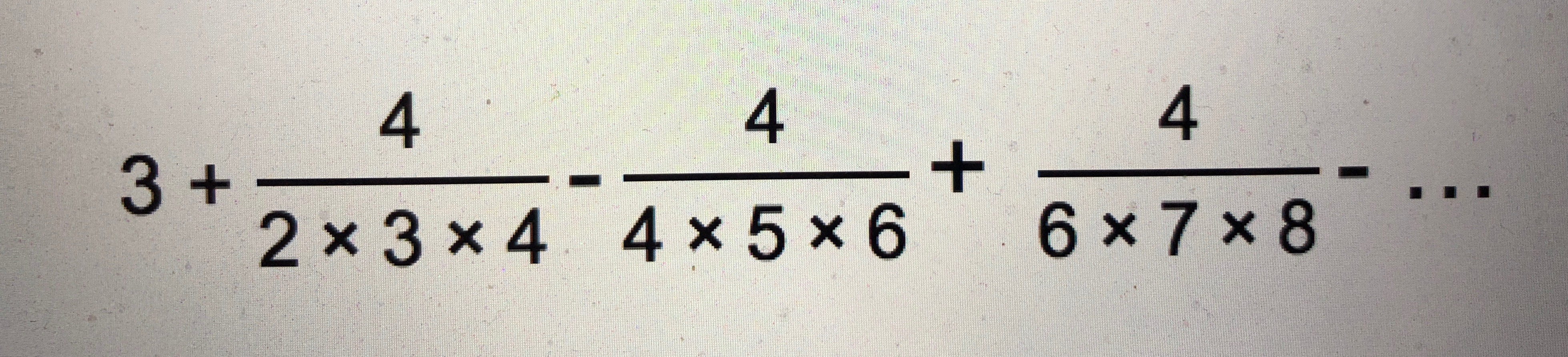• Nursery
• Pre Prep
• Upper School

How I wish I could calculate Pi!

A mathematical challenge!

It is in the Autumn Term of Form 7 that the children first encounter Pi (π) in their Maths lessons. 3.1415926…

Many have already heard of Pi, some even have an idea of how this remarkable irrational value might be used. Some already know that it is irrational and cannot be exactly represented as a simple fraction. No sequence is recurring and it is therefore assumed that any series of numbers could be found within it. As computing power grows, so does our ability to extend the known digits of Pi – the current record is over 22 trillion digits.

They may also know that an approximation of Pi was used by many ancient civilisations, including the Egyptians, Babylonians and Greeks. Their knowledge may even extend to mathematical calculations relating to features in nature.

Form 7 use is limited to two circle applications: calculating circumference and area, where Circumference = 2πr and Area = πr^2. The children use these formulas to calculate the perimeter and area of a variety of circle-related shapes, including semi-circles, quarter circles and annuli. Many children, however, have a curiosity about Pi which extends beyond its limited use in class and sooner or later they will ask, “”How did they (all those historical mathematicians) work out Pi?” So, for all of those minds curious about Pi, and knowing that a little knowledge is a dangerous thing, here are some methods which may be used to find an approximation of Pi.

One method is to measure the circumference (perimeter) of a circle and then divide that by the diameter (the straight line passing from one side of a circle to the other through the centre). Cylinders are quite a good starting place, as they allow for easy measuring of the circumference.

For more accuracy, mathematical methods will be required, such as the method employed by Indian mathematician, Nilakantha Somayaji (1444-1544)The further this calculation is extended, the more accurate the approximation of Pi will be.

And perhaps the most fascinating way is based on Buffon’s needle; a probability approximation which combines the random tossing of toothpicks with a formula.

First divide up a sheet of paper into parallel lines which are the same distance apart as each toothpick is long, i.e. If the toothpicks are 8 cm long, make sure the parallel lines are 8 cm apart. Then randomly throw toothpicks onto the paper: more toothpicks = better results. After tossing, discard any toothpicks which are not completely on the paper then count up all of the remaining toothpicks, and also count up how many sticks are crossing one of the parallel lines.

Finally use this formula to approximate Pi:
Pi = 2 × (total number of toothpicks) ÷ (number of line-crossing toothpicks).

Ultimately, the children will use the π button on their calculators, which stores an approximation to about 10 digits and provides more than ample accuracy for their maths problems. Learning multiple digits of Pi is not essential although if anyone is particularly keen, the current recital record stands at 70 030 digits. If that’s a bit much then count up the letters in each word that makes up the title of this post.

RL

Posted on

Cargilfield where everyday is an adventureWelcome to Cargilfield! We hope this short film gives you a glimpse of what life is like for the girls and boys at our school. We would love to welcome you in person to tour Cargilfield and explain more fully exactly what makes a Cargilfield education so special and so different. Please get in touch with Fiona Craig, our Registrar if you would like to find out more; her email address is [email protected] or you can telephone her on 0131 336 2207.

Don't Show Again